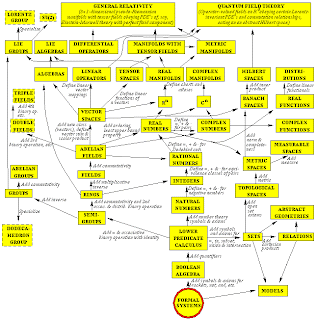## Saturday, March 31, 2007

### Pointless spaceThis is one of my favourite diagrams. Produced by the virtuoso Swedish cosmologist, Max Tegmark, it displays part of the space of all mathematical structures. In particular, it shows which parts of that space are used by general relativity and quantum field theory. To unify relativity and quantum theory it will be necessary, amongst other things, to find a mathematical structure which generalises both of these structures. The obvious candidate already exists: non-commutative geometry. Quantum theory employs non-commutative algebras, relativity employs differential geometry, and non-commutative geometry employs non-commutative differential algebras.

One consequence of this approach is that one obtains an algebraic representation of space, rather than a manifold of spatial points. The conventional manifolds and geometries of relativity can be characterised in terms of the commutative algebra of functions defined upon them. In particular, each point of the underlying manifold corresponds to a special subalgebra called a maximal ideal. In non-commutative geometry, one introduces a non-commutative algebra of functions instead, and then one defines generalisations of the conventional geometrical objects in terms of this non-commutative algebra. There is no underlying manifold of points corresponding to a non-commutative algebra because a non-commutative algebra typically has no maximal ideals.

Whilst the French mathematician Alain Connes has attempted to introduce non-commutative geometry into the standard model of particle physics, Connes's basic construction seems rather ad-hoc to me. I am, however, a big fan of non-commutative geometry as an approach to quantum gravity. Parfionov and Zapatrin give a nice introduction to the idea, and the approach has been pursued in recent years by Michael Heller and his Polish colleagues. Oh, and modesty forbids...